# Thread: Using the method of u-substitution

1. ##Using the method of u-substitution

Using the method of u-substitution,where

u = 3x-7 (enter a function of x)
du = 3 dx (enter a function of x)
a = 2 (enter a number)
b = 5 (enter a number)
f(u) = __________(enter a function of u).

The value of the original integral is____________

so how would i know f(u) and please show steps and explain. so i can understand2. Well, substitute $\displaystyle u$ for $\displaystyle 3x - 7$. You will have a function in terms of $\displaystyle u$. So do that substitution and post what the result is.

3. ???

4. What I was asking you to do is replace $\displaystyle 3x - 7$ with $\displaystyle u$ where ever you see it in the equation.
So,
$\displaystyle \int_3^4\!(u)^4\,dx$
Now, you can almost see $\displaystyle f(u)$, but you need to complete the substitution. If you look at the integral there is still a $\displaystyle dx$ and we need a $\displaystyle du$. So using the substitution
$\displaystyle u = 3x - 7$ we see that
$\displaystyle \frac{du}{dx} = 3 \Rightarrow \frac{1}{3} du = dx$.
Now, make that substitution and reevaluate the limits, i.e. $\displaystyle x=3 \Rightarrow u = 3\cdot3-7=2$ and $\displaystyle x = 4 \Rightarrow u = 3\cdot4 - 7 = 5$
and finally the integral is $\displaystyle \int_2^5\! 3u^4\,du$, so $\displaystyle f(u)=?$

5.so f(u) is 1/3u^4

6. Yes.

Did you understand the steps? When doing u-substitution you will take those steps every time. The hardest part is figuring out what you should use for u.

7. YAY !!!! LOL so, what do i need to do to get: The value of the original integral is____________ << if u can, can u give me a hint or some sort to help for that last answer

8. Evaluate the integral with the u in it just like you would any integral. Find the anti-derivative and then evaluate at the limits.

9. its not clicking in my mind....

10. jpratt,

The value of the original integral is the same value that you found by doing the integral with respect of u as the variable of integration. Can you see why?

11. Also, the crucial thing to try and understand is that finding an anti-derivative (which is virtually your integral) involves working backwards through the chain rule for differentiation. So look at a similar case, a power of a polynomial, but consider differentiation first. Just in case a picture helps...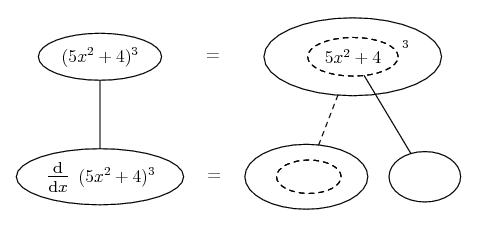Can you differentiate with the chain rule? There's a formula with u, of course, but it amounts to filling out the blanks in this picture, where straight continuous lines differentiate downwards (integrate up) with respect to x, and the straight dashed line similarly but with respect to the dashed balloon expression (the inner function of the composite which is subject to the chain rule).

Spoiler: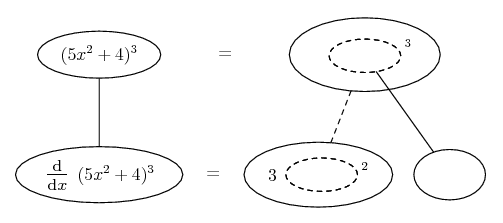Spoiler: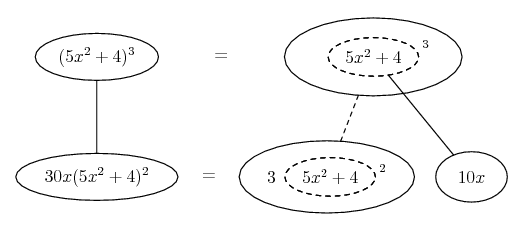To find the anti-derivative (I) for your problem, we should start at the bottom...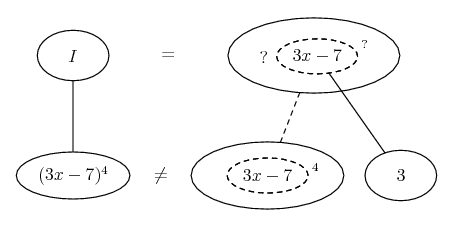... but, bearing in mind the effect of the chain rule coming 'down' (i.e. differentiating), we'll need to cancel the multiplying by 3, in order to have the lower equals sign...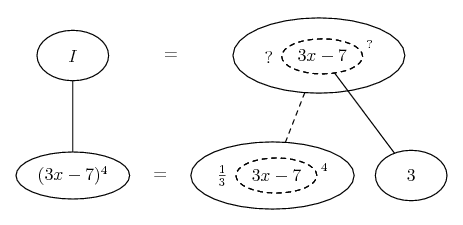Now get an anti-derivative with respect to the dashed balloon...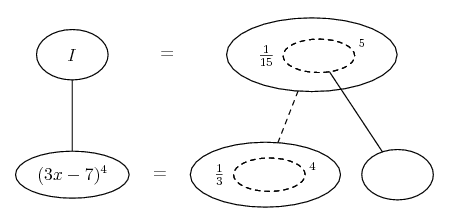Spoiler: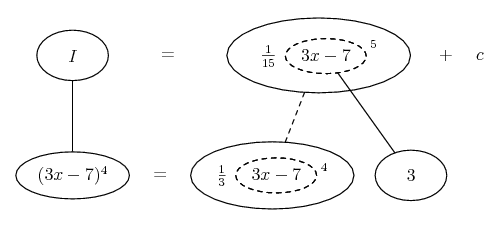At this stage always differentiate your anti-derivative to see if you get your original expression (the 'integrand'). But of course with the picture you can just examine it downwards instead of up.

And then consider the definite integral...

Spoiler: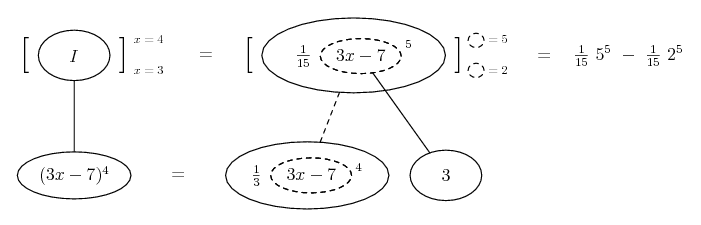_________________________________________

Don't integrate - balloontegrate!

Balloon Calculus; standard integrals, derivatives and methods

Balloon Calculus Drawing with LaTeX and Asymptote!

12. You will learn more techniques to integrate. U-substitution is (probably) the simplest, so make sure you understand it!I red that integrating is harder than differentiating because it is not apparent which technique you need to use. For me I always try to start with the simplest, that being u-substitution.

method, usubstitution